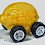## Wednesday, February 25, 2015

### Do the different market models work simultaneously?

Commenter LAL asked if the models (that I fit to empirical data) I presented all together for the first time in this draft of a section  of an eventual paper on information equilibrium could work simultaneously. For most of them, this is actually a trivial direct product since the variables and parameters aren't directly related, except by having a common information source NGDP = N (the IS-LM model has a common 'price' in the interest rate i).

However, there is one potential issue, but it isn't relevant to the post  as presented. In , I used total hours worked (H) as the information receiver (destination) in the labor market, which would have independent parameters from the total employed (L) used in the Solow model. The issue is that one could use L as the receiver in the labor market, which means the model parameters would become a subset of the Solow model and so the models would have to be solved simultaneously. The prices (detectors) in the Solow model weren't used, so we can take p2 to be the price level P without impacting the results.

I drew up a diagram to illustrate the model (aggregate demand, N, in the center as the common information source)  and the potential for conflict between the two models (Solow highlighted in blue and labor in red). The detectors are all shown as labels on the arrows (all of the notations are as in ):

Sure enough, the fits to the Solow model and the labor market model can work simultaneously without adding additional parameters (if you take advantage of the normalization freedom of the price level). Here are the results:

I changed the colors to match up with the diagram above, but otherwise this is the same result. So, yes, the models work simultaneously. Note that I showed here that H and L are in information equilibrium themselves (n.b. the difference in notation where L is E at the link because I used L instead for the civilian labor force), so there is no additional conflict between the two models of the labor market (it turns out information equilibrium -- IE -- is a equivalence relation, so if A in IE with B and B is in IE with C, then A is in IE with C).

#### 1 comment:

1.very interesting